Wohl equation of state

The Wohl equation of state is given by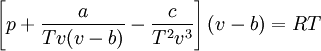$\left[ p + \frac{a}{Tv(v-b)} - \frac{c}{T^2v^3} \right] (v-b) = RT$

where (Eq. 10 in ):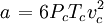$\left. a \right.= 6P_cT_cv_c^2$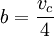$b= \frac{v_c}{4}$

and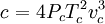$\left. c \right. = 4P_cT_c^2v_c^3$

where$p$ is the pressure,$T$ is the temperature and$R$ is the molar gas constant.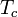$T_c$ is the critical temperature and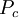$P_c$ is the pressure at the critical point.